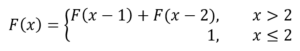## Fibonacci CaseThe Fibonacci numbers are a sequence of integers where the first two elements are 1, and each of the following elements is the sum of the two previous items. This is described mathematically as follows:The first 12 numbers are as follows: 1 1 2 3 5 8 13 21 34 55 89 144.

### Case

Write a loop in UiPath to figure out the first 12 Fibonacci Numbers. The following conditions must be met: